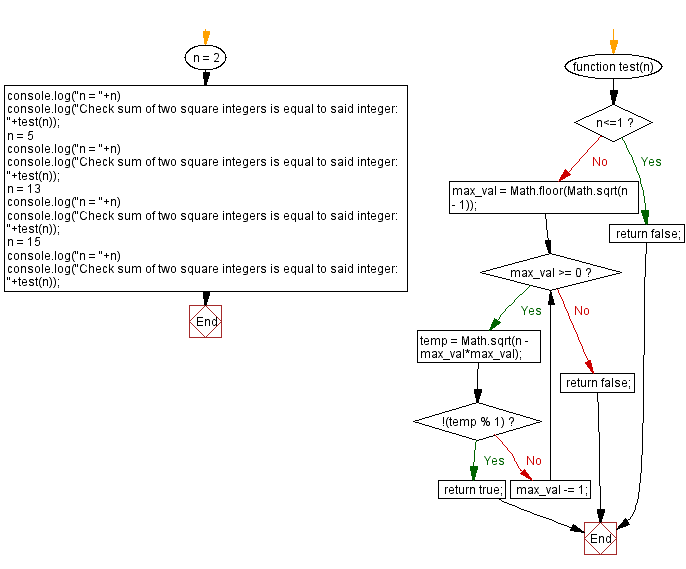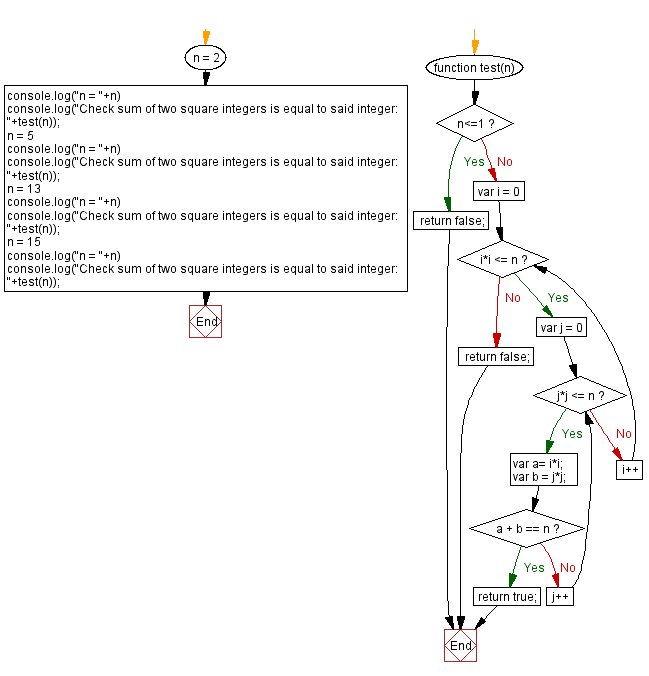# JavaScript: Sum of two square numbers equal to an integer

## JavaScript Math: Exercise-110 with Solution

Write a JavaScript program to check whether the sum of two square integers is equal to another given integer.

For example a2 + b2 = c where c is the given integer.
Example: 13 = 22 + 32

Test Data:
(2) -> true
(5) -> true
(13) -> true
(15) -> false

Sample Solution:

HTML Code:

``````<!DOCTYPE html>
<html>
<meta charset="utf-8">
<title>JavaScript program to Sum of two square numbers equal to an integer</title>
<body>

</body>
</html>
```
```

Solution-1

JavaScript Code:

``````function test(n) {
if (n<=1) return false;
max_val = Math.floor(Math.sqrt(n - 1));
while (max_val >= 0) {
temp = Math.sqrt(n - max_val*max_val);
if (!(temp % 1)) {
return true;
};
max_val -= 1;
};
return false;
}

n = 2
console.log("n = "+n)
console.log("Check sum of two square integers is equal to said integer: "+test(n));
n = 5
console.log("n = "+n)
console.log("Check sum of two square integers is equal to said integer: "+test(n));
n = 13
console.log("n = "+n)
console.log("Check sum of two square integers is equal to said integer: "+test(n));
n = 15
console.log("n = "+n)
console.log("Check sum of two square integers is equal to said integer: "+test(n));
```
```

Sample Output:

```n = 2
Check sum of two square integers is equal to said integer: true
n = 5
Check sum of two square integers is equal to said integer: true
n = 13
Check sum of two square integers is equal to said integer: true
n = 15
Check sum of two square integers is equal to said integer: false
```

Flowchart:Live Demo:

See the Pen javascript-math-exercise-110 by w3resource (@w3resource) on CodePen.

Solution-2

JavaScript Code:

``````function test(n) {
if (n<=1) return false;
for(var i = 0; i*i <= n; i++){
for(var j = 0; j*j <= n; j++)
{
var a= i*i;
var b = j*j;
if(a + b == n)
return true;
}
}
return false;
}

n = 2
console.log("n = "+n)
console.log("Check sum of two square integers is equal to said integer: "+test(n));
n = 5
console.log("n = "+n)
console.log("Check sum of two square integers is equal to said integer: "+test(n));
n = 13
console.log("n = "+n)
console.log("Check sum of two square integers is equal to said integer: "+test(n));
n = 15
console.log("n = "+n)
console.log("Check sum of two square integers is equal to said integer: "+test(n));
```
```

Sample Output:

```n = 2
Check sum of two square integers is equal to said integer: true
n = 5
Check sum of two square integers is equal to said integer: true
n = 13
Check sum of two square integers is equal to said integer: true
n = 15
Check sum of two square integers is equal to said integer: false
```

Flowchart:Live Demo:

See the Pen javascript-math-exercise-110-1 by w3resource (@w3resource) on CodePen.

Improve this sample solution and post your code through Disqus

What is the difficulty level of this exercise?

Test your Programming skills with w3resource's quiz.

﻿